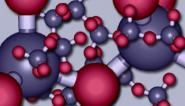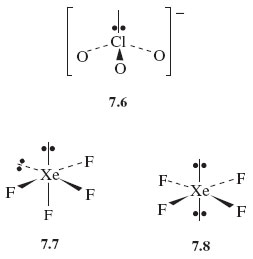Science, Maths & Technology

### Become an OU studentThe molecular world

Start this free course now. Just create an account and sign in. Enrol and complete the course for a free statement of participation or digital badge if available.

# 6.3 Valence-shell electron-pair repulsion theory

The theory of molecular shape that we have been working towards is called valence-shell electron-pair repulsion theory (VSEPR theory). When applied to molecules and ions of the typical elements, its success rate is high. Here is a stepwise procedure that you can follow when applying this theory. It is illustrated with the molecule XeF4 and the ion C1O3. Xenon tetrafluoride is one of the select band of noble gas compounds that were unknown before 1962. The chlorate ion, ClO3, is found in potassium chlorate, KClO3, which is a major ingredient of matches. The steps are as follows:

1 Count the number of outer or valence electrons on the central atom.

Our central atoms are xenon and chlorine, for which the numbers of outer electrons are eight and seven, respectively (Figure 23).

2 If you are dealing with an ion, add one electron for each negative charge and subtract one electron for each positive charge.

XeF4 is a neutral molecule, so the number of valence electrons remains eight. The ion ClO3 carries a single negative charge, so the number of valence electrons is also eight (7 + 1).

3 Assign these electrons to the bonds.

We assume that the bonds formed by the central atom with the halogens or hydrogen are single bonds consisting of one electron pair. Each atom of the bond contributes one electron to this pair. In XeF4, xenon forms four such bonds, so four of its eight outer electrons are used in this way.

At first sight, bonds formed to oxygen are more complicated. Oxygen has six outer electrons, and it can complete its octet in two ways. Both ways are apparent in the structure for ozone (Figure 35c and d). One of the terminal oxygen atoms forms a double bond with the central atom; this bond consists of two shared electron pairs. The other terminal oxygen atom receives a pair of electrons from the central atom, which is the donor in a dative bond. But in both types of bond, the central atom contributes two electrons. Now in C1O3 the central chlorine forms three bonds to oxygens. Six of its eight electrons are therefore used in these bonds.

4 Divide any outer electrons not used in bonding into non-bonded pairs as far as possible.

In XeF4, subtraction of the four xenon bonding electrons from the total of eight leaves four electrons or two non-bonded pairs. In C1O3, subtraction of the six bonding chlorine electrons from the total of eight leaves one non-bonded pair. Each bond, and each non-bonded pair, is now regarded as a repulsion axis, an axis of negative charge that repels other such axes.

5 Count each non-bonded pair and each bond as a repulsion axis. Select the appropriate disposition of these repulsion axes from Figure 47.

The theory assumes that the repulsion axes mimic the bonds formed with fluorine in Figure 47 by getting as far apart as possible. For XeF4, four bonds and two non-bonded pairs give six repulsion axes, and Figure 47 tells us that these will have an octahedral disposition. For C1O3, three bonds and one non-bonded pair give four repulsion axes with a tetrahedral disposition.

6 Choose between any alternative arrangements by minimising inter-axis repulsion.

In many cases, the shape is fully determined at the end of step 5. This is the case for C1O3. We have predicted the shape shown in Structure 7.6, and this suggests that C1O3 should be pyramidal with an inter-bond angle close to the tetrahedral angle of 109.5°. The experimentally observed value is 105°.In some cases, however, a choice between two or more possibilities must be made. XeF4 is one of these. The two non-bonded pairs and four bonds can be octahedrally disposed in two ways (Structures 7.7 and 7.8). It can be quite difficult to choose between such competing arrangements, but a useful procedure assumes that the inter-axis repulsions vary as follows:

non-bonded pair-non-bonded pair > non-bonded pair-bond pair > bond pair-bond pair

The shape can then usually be obtained by choosing that possibility in which the strongest of these different repulsions is minimised. In the case of XeF4, the strongest repulsion is of the non-bonded pair-non-bonded pair type.

## Question 41

Is this type of repulsion lower in Structure 7.7 or Structure 7.8?